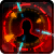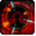# Double tir de précisionConditions

Used by

## Related effects, buffs and debuffs

• [0s] [not displayed]Effect #1

 Slot: Debuff Duration: 0s Tick rate: does not tick # occurrences: 0
• On Apply

Only when the following conditions are met:

• If TARGET does not have 1 or more effects with the tag 8756277914974880926

Perform the following actions:

• Play appearance epp . bounty_hunter . mag_shot . instant_cast, dependent on calling effect
• Weapon Damage
- Is Special Ability = (bool) true
- Ignore Dual Wield Modifier = (bool) true
- Unknown (609) = (bool) false
- Flurry Blows Min = (int) 1
- Flurry Blows Max = (int) 1
- Level Cap = (int) 0
- Threat Percent = (float) 0
- Standard Health Percent Max = (float) 0.428
- Standard Health Percent Min = (float) 0.428
- Amount Modifier Fixed Max = (float) 0
- Amount Modifier Fixed Min = (float) 0
- Amount Modifier Percent = (float) 1.85
- Coefficient = (float) 4.27
- Health Steal Percentage = (float) 0
- Slots = Int(2)
• Weapon Damage
- Is Special Ability = (bool) true
- Ignore Dual Wield Modifier = (bool) false
- Unknown (609) = (bool) false
- Flurry Blows Min = (int) 1
- Flurry Blows Max = (int) 1
- Level Cap = (int) 0
- Threat Percent = (float) 0
- Standard Health Percent Max = (float) 0
- Standard Health Percent Min = (float) 0
- Amount Modifier Fixed Max = (float) 0
- Amount Modifier Fixed Min = (float) 0
- Amount Modifier Percent = (float) 1.85
- Coefficient = (float) 0
- Health Steal Percentage = (float) 0
- Slots = Int(4)
• On Apply

Only when the following conditions are met:

• If TARGET has an effect with the tag 8756277914974880926

Perform the following actions:

• Play appearance epp . bounty_hunter . mag_shot . instant_cast, dependent on calling effect
• Weapon Damage
- Is Special Ability = (bool) true
- Ignore Dual Wield Modifier = (bool) true
- Unknown (609) = (bool) false
- Flurry Blows Min = (int) 1
- Flurry Blows Max = (int) 1
- Level Cap = (int) 0
- Threat Percent = (float) 0
- Standard Health Percent Max = (float) 0.428
- Standard Health Percent Min = (float) 0.428
- Amount Modifier Fixed Max = (float) 0
- Amount Modifier Fixed Min = (float) 0
- Amount Modifier Percent = (float) 1.85
- Coefficient = (float) 4.27
- Health Steal Percentage = (float) 0.25
- Slots = Int(2)
• Weapon Damage
- Is Special Ability = (bool) true
- Ignore Dual Wield Modifier = (bool) false
- Unknown (609) = (bool) false
- Flurry Blows Min = (int) 1
- Flurry Blows Max = (int) 1
- Level Cap = (int) 0
- Threat Percent = (float) 0
- Standard Health Percent Max = (float) 0
- Standard Health Percent Min = (float) 0
- Amount Modifier Fixed Max = (float) 0
- Amount Modifier Fixed Min = (float) 0
- Amount Modifier Percent = (float) 1.85
- Coefficient = (float) 0
- Health Steal Percentage = (float) 0.25
- Slots = Int(4)